# Physics: CBSE Sample Question Paper (2020-21)- 2 Notes | Study Physics Class 12 - NEET

## NEET: Physics: CBSE Sample Question Paper (2020-21)- 2 Notes | Study Physics Class 12 - NEET

The document Physics: CBSE Sample Question Paper (2020-21)- 2 Notes | Study Physics Class 12 - NEET is a part of the NEET Course Physics Class 12.
All you need of NEET at this link: NEET

Class-XII
Physics Theory
TIME: 3 Hrs.
M.M: 70

General Instructions:

(1) All questions are compulsory. There are 33 questions in all.
(2) This question paper has five sections: Section A, Section B, Section C, Section D, and Section E.
(3) Section A contains ten very short answer questions and four assertion reasoning MCQs of 1 mark each, Section B has two case-based questions of 4 marks each, Section C contains nine short answer questions of 2 marks each, Section D contains five short answer questions of 3 marks each and Section E contains three long answer questions of 5 marks each.
(4) There is no overall choice. However, an internal choice is provided. You have to attempt only one of the choices in such questions.

Section A

All questions are compulsory. In case of internal choices, attempt any one of them.
Q. 1. What is the ratio of contributions made by the electric field and magnetic field
components to an EM wave's intensity?   (1 Mark)
Ans: Average energy by electric field E0 is UAV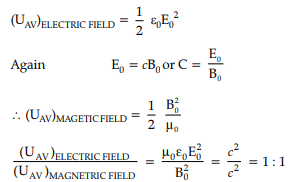Q.2. An electron is moving with an initial velocity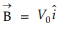in a magnetic field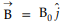. What is its de-Broglie wavelength?   (1 Mark)
Ans: Given,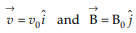Magnetic force on moving electron =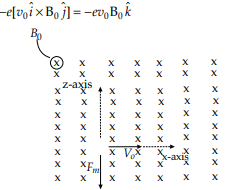So, the force is perpendicular to v and B, both as the force is perpendicular to the velocity.
So, the magnitude will not change v or mv (p = mv momentum) so, the de-Broglie
wavelength remains the same.

OR

Which optical instrument is used to see an erect image of a distant object?  (1 Mark)
Ans: Reflecting telescope is used to see an erect image of a distant object.

Q.3. Consider a current carrying wire (current I) in the shape of a circle. Note that as the current progresses along the wire, the direction of j (current density) changes in an exact manner, while the current I remain unaffected. Which agent is essentially responsible for this?    (1 Mark)
Ans: Electric field produced by charges accumulated on the surface of the wire can be
varied.

Q.4. What type of resistance is to be added with a galvanometer in which combination for it to act as a voltmeter?   (1 Mark)
Ans: To work as a voltmeter, the resistance of the galvanometer should be high. A voltmeter indeed is a modified form of a pivoted coil galvanometer. Since the coil's resistance of the galvanometer of its own is low, hence to convert a galvanometer into a voltmeter, its resistance is to be increased. For this, an appropriate high resistance is joined in series with the galvanometer.

OR

Why are microwaves considered suitable for radar systems used in aircraft navigation?   (1 Mark)
Ans: Microwaves are considered suitable for radar systems used in aircraft navigation because of their short wavelength range which makes them suitable for long-distance communication.

Q.5. On moving a charge of 20 C by 2 cm, 2 J of work is done; what is the potential difference between the points?  (1 Mark)
Ans: The electric potential at any point due to a charge Q is given by,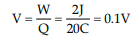Q.6. Light with an energy flux of 20 W/cm2 falls on a non-reflecting surface at
normal incidence. If the surface has an area of 30 cm2 , what will be the total
momentum delivered (for complete absorption) during 30 minutes?   (1 Mark)
Ans: Energy flux =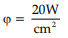Area A = 30 cm2 ,
time t =30 × 60
U = Total energy falling in t S = Energy flux × Area × time = ϕAt
U = 20 × 30 × 30 × 60 J
Momentum of the incident light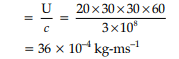For no reflection and complete absorption momentum of reflected radiation is zero. Momentum delivered to surface = Change in momentum
= Pf – P= 0 – 36 × 10–4 kg-ms–1
= – 36 × 10–4 kg-ms–1
(-) sign shows the direction of momentum.

Q.7. Three long, straight parallel wires carrying current are arranged as shown in
the figure.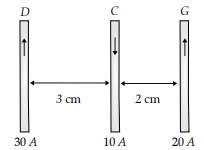What will be the force experienced by a 25 cm length of wire C ?   (1 Mark)
Ans: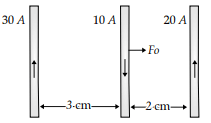Force on wire C due to wire D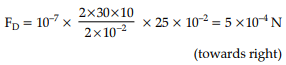Force on wire C due to wire G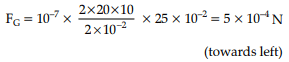So, the net force of wire C
FNET = F– FG = 0.

OR

Four nuclei of an element undergo fusion to form a heavier nucleus, with the release of energy. Which of the two — the parent or the daughter nuclei — would have higher binding energy per nucleon?   (1 Mark)
Ans: Daughter nuclei will have higher binding energy per nucleon. The mass of a heavier
nucleus (daughter) is less than the sum of masses of combining nuclei. So, the mass defect is more in daughter nuclei resulting in more binding energy per nucleon.

Q.8. An iron-cored solenoid has self-inductance 2.8 H. When the core is removed,
the self inductance becomes 2 mH. What is the relative permeability of the core
used?    (1 Mark)
Ans: Relative permeability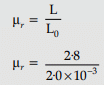= 1400

OR

The electric potential is constant in a given region. What will be the nature of the electric field in that region?  (1 Mark)
Ans: Electric field = E = – dV/dx
When V is constant, then dV/dx = 0
Therefore, Electric field = E = 0

Q.9. In which state do the set of atoms in an excited state decay?  (1 Mark)
Ans: A set of atoms in an excited state decays in general to any of the states with lower energy.

Q.10. Write the two processes involved in the formation of p-n junction.  (1 Mark)
Ans: The two processes those take place in the formation of a p-n junction are :
(i) Diffusion
(ii) Drift
1. When a p-n junction is being formed, holes diffuse from the p-side to the n-side and
electrons diffuse from n-side to the p-side due to the concentration gradient of holes and
electrons.
2. An electric field develops across the p-n junction, this electric field causes the
movement of electrons from the p-side to the n-side and holes from the n-side to p-side.
This motion of charged carrier due to the electric field is called drift.

For question numbers 11, 12, 13 and 14, two statements are given-one labelled Assertion (A) and the other labelled Reason (R). Select the correct answer to these questions from the codes (a), (b), (c) and (d) as given below:
Q.11. Assertion (A): Magnetic field interacts with a moving charge only.
Reason (R): Moving charge produces a magnetic field.  (1 Mark)
(a) Both A and R are true, and R is the correct explanation of A
(b) Both A and R are true, but R is NOT the correct explanation of A
(c) A is true, but R is false
(d) A is false, and R is also false
Ans: Correct option is (a)
Explanation: Current carrying wire creates a magnetic field. This magnetic field has no effect on a stationary charge. But when the charge also moves, then it creates a current. This current produces a magnetic field. Two fields interact and the charge is deflected. So, the assertion is true.
Moving charge creates a current which produces a magnetic field. So, the reason is also true. The reason is the correct explanation of A.

Q.12. Assertion (A): The Coulomb force is the dominating force in the universe.
Reason (R): Coulomb force is weaker than the gravitational force.  (1 Mark)
(a) Both A and R are true, and R is the correct explanation of A
(b) Both A and R are true, but R is NOT the correct explanation of A
(c) A is true, but R is false
(d) A is false, and R is also false
Ans: Correct option is (d)
Explanation: Gravitational force is the dominating force in the universe. So assertion is
false.
Gravitational force is weaker than the Coulomb force. So, the reason is also false.

Q.13. Assertion (A): The magnetic field configuration with 3 poles is not possible.
Reason (R): No torque acts on a bar magnet itself due to its own field.  (1 Mark)
(a) Both A and R are true, and R is the correct explanation of A
(b) Both A and R are true, but R is NOT the correct explanation of A
(c) A is true, but R is false
(d) A is false, and R is also false
Ans: Correct option is (b)
Explanation: Magnetic poles exist in pairs. So the assertion is true.
The bar magnet does not exert a torque on itself in its own magnetic field. Torque
is proportional to cross product of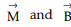. The angle between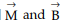being 0,
the cross product is 0. So, there will be no torque. So the reason is also true. But R cannot explain A.

Q.14. Assertion (A): Einstein demonstrated the wave nature of light by
photoelectric effect.
Reason (R): Number of photo electrons emitted is proportional to the frequency of incident light.  (1 Mark)
(a) Both A and R are true, and R is the correct explanation of A
(b) Both A and R are true, but R is NOT the correct explanation of A
(c) A is true, but R is false
(d) A is false, and R is also false
Ans: Correct option is (d)
Explanation: Photoelectric effect demonstrates the particle nature of light. So, the assertion is false.
Number of photoelectrons emitted is proportional to the intensity of incident light. So, the reason is false. Both A and R are false.

Section B

Questions 15 and 16 are Case Study based questions and are compulsory. Attempt any 4 subparts from each question. Each question carries 1 mark.
Q.15. Photometry: The measurement of light as perceived by the human eye is called
photometry. Photometry is a measurement of a physiological phenomenon, being the
stimulus of light as received by the human eye, transmitted by the optic nerves and
analysed by the brain. The main physical quantities in photometry are (i) the luminous
intensity of the source, (ii) the luminous flux or flow of light from the source, and (iii)
the illuminance of the surface. The SI unit of luminous intensity (I) is candela (cd). The
candela is the luminous intensity, in a given direction, of a source that emits
monochromatic radiation of frequency 540 × 1012 Hz and that has a radiant intensity in
that direction of 1/683 watt per steradian. If a light source emits one candela of luminous
intensity into a solid angle of one steradian, the total luminous flux emitted into that solid
angle is one lumen (lm). A standard 100 watt incandescent light bulb emits approximately
1700 lumens.

1. What is photometry?  (1 Mark)
(a) Measurement of light as perceived by the human eye
(b) Measurement of number of photons emerging from a light source
(c) Measurement of electrons emitted by photosensitive surface
(d) Measurement of photosensitivity
Ans: Correct option is (a)
Explanation: The measurement of light as perceived by the human eye is called photometry.
2. Light received by the human eye is analysed by  (1 Mark)
(a) Retina
(b) brain
(c) optic nerve
(d) Nervous system
Ans: Correct option is (b)
Explanation: Light received by the human eye, transmitted by the optic nerves and
analysed by the brain.

3. The SI unit of luminous intensity is  (1 Mark)
(a) Diopter
(c) Candela
(d) Lumen
Ans: Correct option is (c)
Explanation: The SI unit of luminous intensity (I) is candela (cd).

4. Unit of luminous flux is  (1 Mark)
(a) Candela
(c) Nit
(d) Lumen
Ans: Correct option is (d)
Explanation: The total luminous flux emitted into a solid angle is one lumen (lm).

5. A standard 100-watt incandescent light bulb emits approximately  (1 Mark)
(a) 1700 Lumen
(b) 700 Lumen
(c) 1200 Lumen
(d) 1000 Lumen
Ans: Correct option is (a)
Explanation: A standard 100-watt incandescent light bulb emits approximately 1700 lumens.

Q.16. It is a heavily doped pn junction which under forward bias emits spontaneous
radiation. The diode is encapsulated with a transparent cover so that emitted light can come out. When the diode is forward biased, electrons are sent from n → p (where they are minority carriers) and holes are sent from p → n (where they are minority carriers). At the junction boundary, the concentration of minority carriers increases as compared to the equilibrium concentration (i.e., when there is no bias). Thus at the junction boundary on either side of the junction, excess minority carriers are there which recombine with majority carriers near the junction. On recombination, the energy is released in the form of photons. Photons with energy equal to or slightly less than the band gap are emitted. When the forward current of the diode is small, the intensity of light emitted is small. As the forward current increases, intensity of light increases and reaches a maximum. Further increase in the forward current results in decrease of light intensity. LED's are biased such that the light emitting efficiency is maximum.
The V-I characteristics of a LED is similar to that of a Si junction diode. But, the threshold voltages are much higher and slightly different for each colour. The reverse breakdown voltages of LED's are very low, typically around 5V. So care should be taken that high reverse voltages do not appear across them. LED's that can emit red, yellow, orange, green and blue light are commercially available :

1. LED is a:   (1 Mark)
(a) Lightly doped p-n junction diode
(b) Heavily doped p-n junction diode
(c) Moderately doped p-n junction diode
(d) Two back to back p-n junction diode
Ans: Correct option is (b)
Explanation: LED is a heavily doped p-n junction diode.

2. LED emits light:   (1 Mark)
(a) When reversed biased
(b) When forward biased
(c) When forward or reverse biased
(d) When heated
Ans: Correct option is (b)
Explanation: LED under forward bias emits spontaneous radiation.

3. During recombination at the junction, emitted photons have:   (1 Mark)
(a) energy greater than the band gap
(b) energy equal to or slightly less than the band gap
(c) energy which has no relation with the band gap
(d) very low energy compared to band gap
Ans: Correct option is (b)
Explanation: On recombination, the energy is released in the form of photons. Photons
with energy equal to or slightly less than the band gap are emitted.

4. Threshold voltage of LED is:   (1 Mark)
(a) Lower compared to other p-n junction diodes and slightly different for each colour
(b) Higher compared to other p-n junction diodes and slightly different for each colour
(c) Higher compared to other p-n junction diodes and same for all colours
(d) Lower compared to other p-n junction diodes and same for all colours
Ans: Correct option is (b)
Explanation: The V-I characteristics of a LED is similar to that of a Si junction diode. But
the threshold voltages are much higher and slightly different for each colour.

5. The reverse breakdown voltages of LED's are:   (1 Mark)
(a) Very low and typically around 0.5V
(b) Very low and typically around 5V
(c) Very high and typically around 50V
(d) Very low and typically around 0.05V
Ans: Correct option is (b)
Explanation: The reverse breakdown voltages of LED's are very low, typically around 5V.

Section C
All questions are compulsory. In the case of internal choices, attempt anyone.
Q.17. (i) State Biot-Savart's law in vector form, expressing the magnetic field due to an element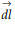carrying current I at a distance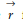from the element.
(ii) Write the expression for the magnitude of the magnetic field at the centre of a circular loop of radius r carrying a steady current I. Draw the magnetic field lines due to the current loop.   (2 Mark)
Ans: (i) According to Biot-Savart’s law, the magnetic field due to a current element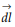carrying current I at a point P with position vector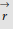is given by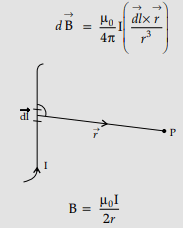(ii) Field lines :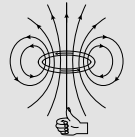Q.18. State Kirchhoff's rules. Explain briefly how these rules are justified.  (2 Mark)
Ans: Kirchhoff’s first law is known as junction rule which states that for a given junction or node in a circuit, sum of the currents entering will be equal to sum of currents leaving.
Kirchhoff’s second law is also known as loop rule which shows that around any closed loop in a circuit, sum of the potential differences across all elements will be zero.
Justification: The junction rule is in accordance with the conservation of charge that serves as basis of current rule while loop rule is based on law of conservation of energy.
Kirchhoff's first law: The sum of the currents flowing towards a junction or node is equal to the sum of currents leaving the junction or node. If I1, I2 and I3 are the currents towards a node. I4 and I5 are the currents leaving the node.
According to Kirchhoff’s 1st law: I1 + I2 + I3 − I4 − I= 0
i.e. SI = 0
Kirchhoff's second Law: The algebraic sum of all potential drops and e.m.f.s in a closed path or loop is zero i.e. SE = SIR Kirchhoff’s 1st law is in accordance with the
conservation of charge. Kirchhoff’s 2nd law is in accordance with the conservation of
energy.

OR

Five charges, q each are placed at the corners of a regular pentagon of side 'a'.
(i) What will be the electric field at O if the charge from one of the corners (say A) is removed?
(ii) what will be the electric field at O if the charge q at A is replaced by – q?  (2 Mark)
Ans: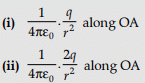(i) If a charge q is removed from point A, a negative change is developed at A where electric field will be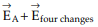= 0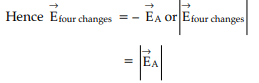When change q is removed from A, net electric field at the centre due to remaining
charges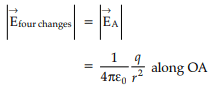(ii) If a charge q is replaced by charge–q at point A, this generates a net electric field at point O as a result of – 2q change, so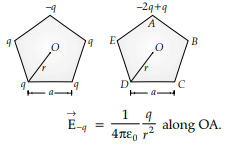Hence net electric field at the centre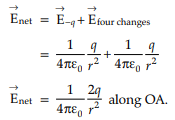Q.19. A light bulb and a solenoid are connected in series across an ac source of voltage.
Explain, how the glow of the light bulb will be affected when an iron rod is inserted in the solenoid.   (2 Mark)
Ans:
Explanation: Self-inductance of solenoid increases; this increases the impedance of the circuit and hence current decreases.
Detailed Answer: (i) Brightness will decrease when an iron rod is inserted in the solenoid.
(ii) When an iron rod is inserted in the solenoid, the iron rod will cut the magnetic field lines of an inductor, so as per Kirchhoff’s and Faraday’s Laws, cutting of magnetic field will tend to induce a current inside the inductor which opposes the direction of it’s the cause. So when the current is being induced by the moving rod, it opposes the flow of existing current in the circuit, causing the bulb’s brightness to go down as there is less current passing through it.

OR

Professor C.V. Raman surprised his students by suspending a tiny light ball freely
in a transparent vacuum chamber by shining a laser beam on it. Which property of
EM waves was he exhibiting? Give one more example of this property?  (2 Mark)
Ans: The Properties of electromagnetic waves are similar to other waves as it carries both energy and momentum due to which it exerts radiation pressure. A tiny ball can be
suspended freely in a transparent vacuum chamber by shining a laser beam on it as an electromagnetic wave exerts radiation pressure. According to him, the tails of the comets are due to radiation pressure.

Q.20. A long straight current-carrying wire passes normally through the centre of circular loop. If the current through the wire increases, will there be an induced emf in the loop? Justify.  (2 Mark)
Ans: No, As the magnetic field due to the current-carrying wire will be in the plane of the circular loop, so magnetic flux will remain zero.
Alternatively, [Magnetic flux does not change with the change of current.]
Detailed Answer: No, there will not be an induced emf in the loop as magnetic flux doesn’t change linked with the circular loop because magnetic field lines are parallel to the plane of the loop.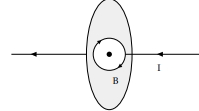As induced emf (e) is proportional to the rate of change of magnetic flux (ϕB) and ϕB = B·A = BAcos θ
Here, B⊥A ⇨ ϕB = BAcos 90° = 0
So, induced emf = 0. Hence, a change in current will not create any emf in the loop.

Q.21. If the light of wavelength 412.5 nm is incident on each of the metals given below, which one of the following will show photoelectric emission and why?  (2 Mark)

 Metal Work Function (eV) Na 1.92 K 2.15 Ca 3.20 Mo 4.17

Ans: The energy of a photon of incident radiation is given by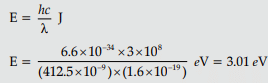Hence, only Na and K will show photoelectric emission.
[Note: Award this ½ mark even if the student writes the name of only one of these metals]
Reason: The energy of the incident photon is more than the work function of only these two metals.
Detailed Answer: Energy of incident photon : The energy of a photon of incident radiation is given by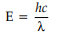Where h = Planck’s constant = 6.63 × 10–34 Js
c = velocity of light = 3 × 108 ms–1
λ = Wavelength = 412.5 nm = 412.5 × 10–9 m Putting the values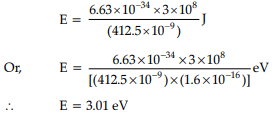Photoelectric emission will be shown by Na and K only.
Since the energy of incident Photon is greater than the work functions of Na and K,
photoelectric emission will take place from those two ,metals only.

Q.22. A square loop of side 20 cm carrying current of 1 A is kept near an infinite long straight wire carrying a current of 2 A in the same plane as shown in the
figure.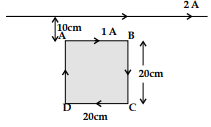Calculate the magnitude and direction of the net force exerted on the loop due to the current carrying conductor.    (2 Mark)
Ans: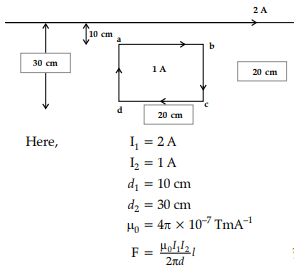Net force on AB and CD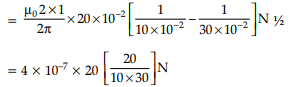= 5.33 × 10–7 N
This net force is directed towards the infinitely long straight wire.
Net force on sides BC and DA = zero. ∴ Net force on the loop = 5.33 × 10–7 N
The force is directed towards the infinitely long straight wire.

Q.23. An α-particle and a proton are accelerated through the same potential. Find the ratio of their de-broglie wavelengths. (2 Mark)
Ans: de-Broglie wavelength is given by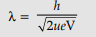Mass of a proton = u
Charge of proton = e
Hence, Kinetic energy of a proton when accelerated through a potential = eV
Putting these values in de-Broglie wavelength formula to calculate wave length of proton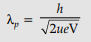Now,
• Mass of an a-particle = 4u
• Charge of an a-particle = 2e
Hence, Kinetic energy of an a-particle when accelerated through a potential =2 eV
Putting these values in de-Broglie wavelength formula to calculate wave length of a-particle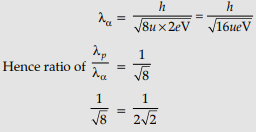Q.24. Distinguish between ‘intrinsic’ and ‘extrinsic’ semiconductors.  (2 Mark)
Ans: Differences:

 Intrinsic Extrinsic (i) Pure semiconductor Doped with impurity (ii) Low conductivity to room temperature. Higher conductivity at room temperature. (iii) Conductivity depends on temperature Conductivity does not depend significantly on temperature.

OR

A square-shaped plane coil of area 100 cm2 of 200 turns carries a steady current of 5 A. It is placed in a uniform magnetic field of 0.2 T acting perpendicular to the plane of the coil. Calculate the torque on the coil when its plane makes an angle of 60° with the direction of the field. In which orientation will the coil be in stable equilibrium?  (2 Mark)
Ans: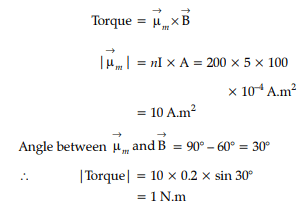Q.25. Assuming that the two diodes Dand D2 used in the electric circuit shown in the figure are ideal, find out the value of the current flowing through 2.5 Ω resistor.  (2 Mark)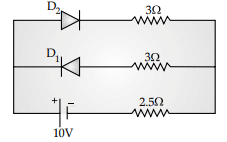Ans: In the circuit, if D1 is open and Dis short then equivalent circuit will result as :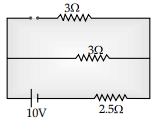D2 is reverse biased
∴ D1 conducts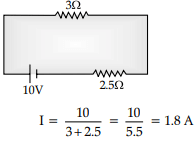Section D

All questions are compulsory. In case of internal choices, attempt anyone.
Q.26. (a) Derive an expression for the velocity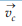of a positive ion passing undeflected through a region where crossed and uniform electric field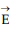and magnetic field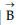are simultaneously present.
(b) Draw and justify the trajectory of identical positive ion whose velocity has a magnitude less than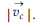(3 Mark)
Ans: (a)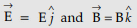Force on positive ion due to electric field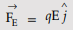Force due to magnetic field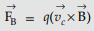For passing undeflected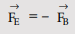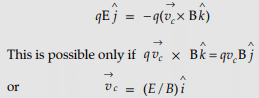(b) The trajectory would be as shown below: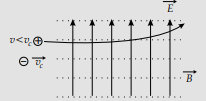Justification: For positive ions with speed v < vc
Force due to electric field = F'E = qE = F
due to magnetic field F' B = qvB < FB
since v < vc
Now forces are unbalanced, and hence, the ion will experience an acceleration along E.
Since initial velocity is perpendicular to E, the trajectory would be parabolic.

Q.27. Derive the expression for the current density of a conductor in terms of the conductivity and applied electric field. Explain, with reason how the mobility of electrons in a conductor changes when the potential difference applied is doubled, keeping the temperature of the conductor constant.  (3 Mark)
Ans: Derivation of current density explains with reason, the change in mobility of electrons.
Using Ohm's law,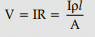Potential difference (V), across the ends of a conductor of length 'l', where field 'E' is applied is given by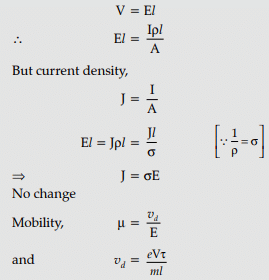As the potential is doubled, drift velocity also gets doubled, therefore, no change in mobility.

OR

(a) Name the device used to change the alternating voltage to a higher or lower value. State one cause for power dissipation in this device.
(b) Explain with an example, how power loss is reduced if the energy is transmitted over long distances as an alternating current rather than a direct current.  (3 Mark)
Ans: (a) Transformer is used to change the alternating voltage to a higher or lower value.
One cause for power dissipation in a transformer is the resistance of the copper wire winding. This resistance causes I2 R loss in form of heat.
(b) AC can be stepped up by using a transformer but DC cannot be stepped up.
As power remains constant when AC is stepped up, voltage increases but the current decreases. Decreases in current reduce I2 R loss. So, while transmitting AC, power loss
can be thus reduced but for transmission of DC, power loss cannot be reduced.

Q.28. A bar magnet of magnetic moment 6 J/T is aligned at 60° with a uniform external magnetic field of 0.44 T.
Calculate: (a) the work done in turning the magnet to align its magnetic moment
(i) normal to the magnetic field,
(ii) opposite to the magnetic field, and
(b) the torque on the magnet in the final orientation in case (ii).  (3 Mark)
Ans: (a) Work done = mB (cos θ1 − cos θ2)
(i) θ1 = 60°, θ2 = 90°
∴ work done    = mB(cos 60°− cos 90°)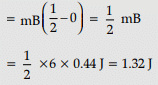(ii) θ1 = 60°, θ2 = 180°
∴ work done    = mB(cos 60°− cos 90°)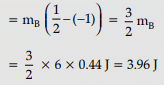[Also accept calculations done through changes in potential energy.]
(b) Torque =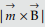= mB sin θ
For θ = 180°, we have
Torque = 6×0.44 sin 180° = 0

OR

A source of ac voltage V = V0sin ωt, is connected across a pure inductor of
inductance L. Derive the expressions for the instantaneous current in the circuit.
Show that average power dissipated in the circuit is zero.   (3 Mark)
Ans: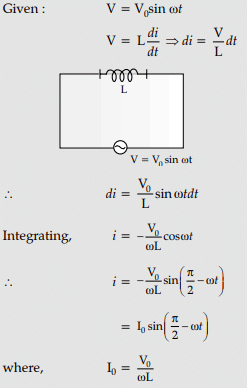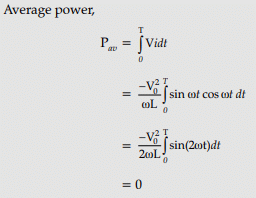Q.29. Define mutual inductance between a pair of coils. Derive an expression for the mutual inductance of two long coaxial solenoids of same length wound one over the other.  (3 Mark)
Ans: (i) Definition of mutual inductance: Mutual inductance, is numerically equal to the induced emf in the secondary coil when the current in the primary coil changes by unity.
Alternatively, Mutual inductance in numerically equal to the magnetic flux linked with one coil/secondary coil when unit current flows through the other coil/primary coil.
(ii) Derivation of mutual inductance for two long solenoids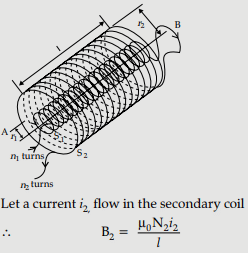∴ Flux linked with the primary coil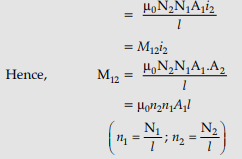Q.30. Show that in the free oscillations of an LC circuit, the sum of energies stored in the capacitor and the inductor is constant in time.  (3 Mark)
Ans: At an instant t, change q on the capacitor and the current i are given by :
q(t) = q0 cos ωt
i(t) = – q0ω sin wt
Energy stored in the capacitor at time t is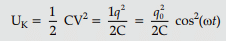Energy stored in the inductor at time t is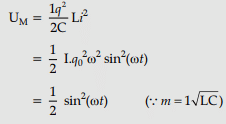Sum of energies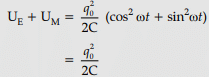The sum is constant in time as q0 and C both are time-independent.

Section E

All questions are compulsory. In case of internal choices, attempt any one.
Q.31. (i) Draw a labelled diagram of a step-up transformer. Obtain the ratio of secondary to primary voltage in terms of number of turns and currents in the two coils.
(ii) A power transmission line feeds input power at 2200 V to a step-down transformer with its primary windings having 3000 turns. Find the number of turns in the secondary to get the power output at 220 V.   (5 Mark)
Ans: (i) Labelled diagram of a step-up transformer.
Derivation of ratio of secondary and primary voltage.
(ii) Calculation of number of turns in the secondary.
(i)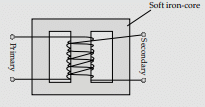Working principle: Whenever current in one coil changes, an emf gets induced in the neighbouring coil
Voltage across secondary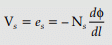Voltage across primary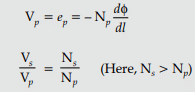In an ideal transformer,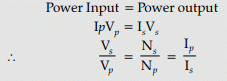(ii)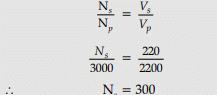Q.32. (i) Write three observed features of photoelectric effect which cannot be explained by wave theory of light. Explain how Einstein’s photoelectric equation is used to describe these features satisfactorily.
(ii) Figure shows a plot of stopping potential (V0) with frequency (ν) of incident radiation for two photosensitive materials M1 and M2
Explain: (a) Why the slope of both the lines is same?
(b) For which material emitted electrons have greater kinetic energy for the same frequency of incident radiation?   (5 Mark)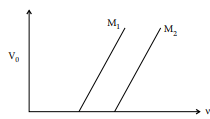Ans: (i) Three features of photoelectric effect, which cannot be explained by the wave theory of light, are :
(a) Maximum kinetic energy of emitted electrons is independent of the intensity of incident light.
(b) There exists a ‘threshold frequency’ for each photosensitive material.
(c) ‘Photoelectric effect’ is instantaneous in nature.
Einstein’s photoelectric equation K.E.max= hv – ϕ0
[Alternatively: eV0 = hv – ϕ0 ] can be used to explain these features as follows.
(a) Einstein’s equation shows that Kmax∝ v. However, Kmax does not depend on the intensity of light.
(b) Einstein’s equation shows that for v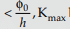becomes negative, i.e, there cannot be any photoemission for v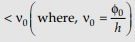.
(c) The free electrons in the metal, that completely absorb the energy of the incident photons, get emitted instantaneously.
(ii) (a) Slope of the graph between V0 and n (from Einstein’s equation) equals (h/e). Hence, it does not depend on the nature of the material.
(b) Emitted electrons have greater energy for material M1. This is because ϕ0 (= hv0) has a lower value for material M1

OR

(i) Use Gauss’s law to derive the expression for the electric field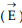due to a straight uniformly charged infinite line of charge density λ C/m.
(ii) Draw a graph to show the variation of E with perpendicular distance r from line of charge.
(iii) Find the work done in bringing a charge q from perpendicular distance r1 to r2 (r2 > r1).   (5 Mark)
Ans: (i) Derivation of the expression for electric field
(ii) Graph to show the required variation of the electric field
(iii) Calculation of work done
(i)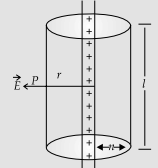To calculate the electric field, imagine a cylindrical Gaussian surface, since the field is everywhere radial, flux through two ends of the cylindrical Gaussian surface is zero.
At cylindrical part of the surface electric field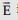is normal to the surface at every point and its magnitude is constant.
Therefore flux through the Gaussian surface = Flux through the curved cylindrical part of the surface,
= E × 2prl   ...(i)
Applying Gauss's Law
Flux,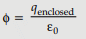Total charge enclosed = Linear charge density × l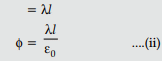Using Equations (i) & (ii)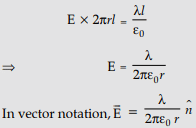(where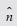is a unit vector normal to the line charge)
(ii) The required graph is as shown :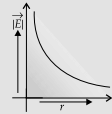(iii)Work done in moving the charge 'q' through a small displacement 'dr’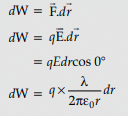Work done in moving the given charge from r1 to r2 (r2 > r1)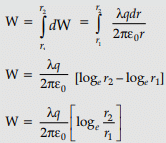Q.33. A device ‘X’ is connected to an ac source V = V0sin ωt. The variation of voltage, current and power in one cycle is shown in the following graph :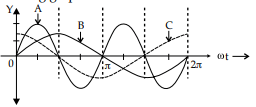(i) Identify the device ‘X’.
(ii) Which of the curves A, B and C represent the voltage, current and the power consumed in the circuit ? Justify your answer.
(iii) how does its impedance vary with frequency of the ac source ? Show graphically.
(iv) Obtain an expression for the current in the circuit and its phase relation with ac voltage.  (5 Mark)
Ans: (i) Identification
(ii) Identifying the curves and justification
(iii) Variation of Impedance with frequency and Graph
(iv) Expression for current and Phase relation
Detailed Answer: (i) The device X is a capacitor.
(ii) Curve B → voltage
Curve C → current
Curve A → power
Reason: The current leads the voltage in phase, by π/2 , for a capacitor.
(iii)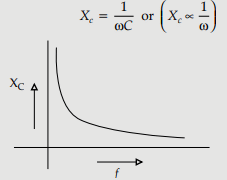(iv) V = V0sin ωt
q = VC = CV0sin ωt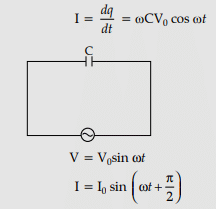Current leads the voltage, in phase, by π/2
[Note: If the student identifies the device X as an Inductor but writes a correct answer to parts (c) and (d) (in terms of an inductor), the student will be given full marks for (only) these two parts]

OR

(a) Draw a labeled diagram of a moving coil galvanometer. Describe briefly its principle and working.
(i) Why is it necessary to introduce a cylindrical soft iron core inside the coil of a galvanometer?
(ii) Increasing the current sensitivity of a galvanometer may not necessarily increase its voltage sensitivity. Explain, giving a reason.  (5 Mark)
Ans: (a)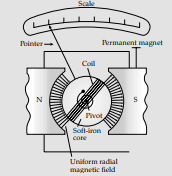Principle and working : A current carrying coil, placed in a uniform magnetic field, can experience a torque.
Consider a rectangular coil for which no. of turns = N,
Area of cross-section = l × b = A,
Intensity of the uniform magnetic field = B,
Current through the coil = I
∴ Deflecting torque = BIl × b = BIA
For N turns, t = NBIA
Restoring torque in the spring = kθ (k = restoring torque per unit twist)
∴ NBIA = kθ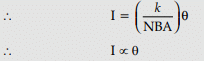The deflection of the coil is therefore, proportional to the current flowing through it.
(b) (i) The soft iron core not only makes the field radial but also increases the strength of the magnetic field.
(ii) We have Current sensitivity =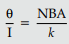Voltage sensitivity =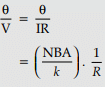It follows that an increase in current sensitivity may not necessarily increase the voltage sensitivity.

The document Physics: CBSE Sample Question Paper (2020-21)- 2 Notes | Study Physics Class 12 - NEET is a part of the NEET Course Physics Class 12.
All you need of NEET at this link: NEETUse Code STAYHOME200 and get INR 200 additional OFF

## Physics Class 12

134 videos|388 docs|213 tests

### How to Prepare for NEET

Read our guide to prepare for NEET which is created by Toppers & the best Teachers

Track your progress, build streaks, highlight & save important lessons and more!

,

,

,

,

,

,

,

,

,

,

,

,

,

,

,

,

,

,

,

,

,

;